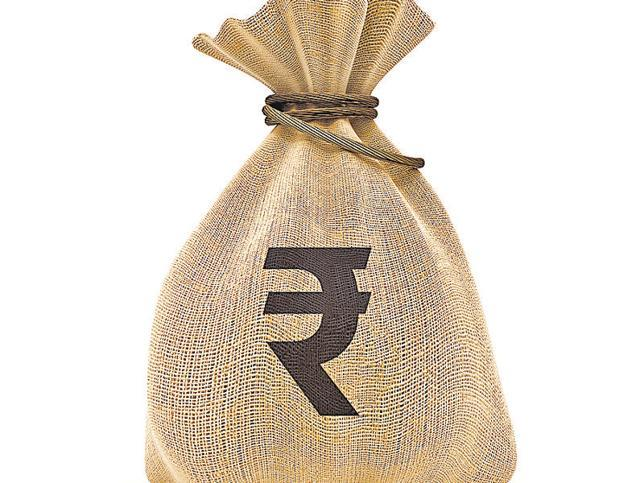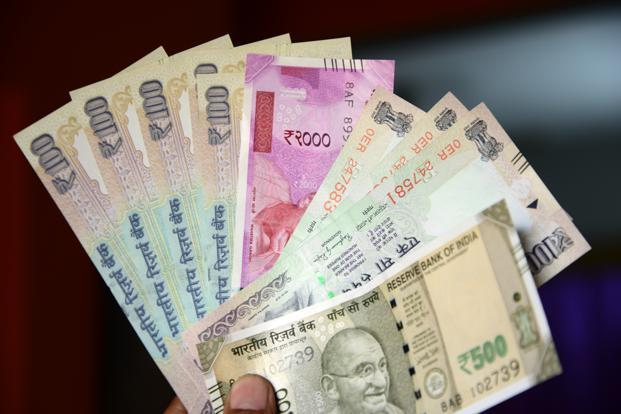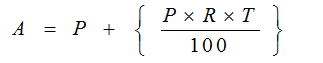# Arithmetic

#### Simple Interest & Compound Interest - 1

Simple Interest & Compound Interest - 1

Simple Interest/ Compound Interest

Simple InterestThis chapter covers the basics of simple interest, and the purpose of this chapter is to help you understand what exactly the meaning of simple interest is. When an amount is borrowed from, we agree to pay it back with some additional charges, which is known as Simple Interest. This lesson will teach you how to calculate Simple Interest.

What does Interest actually mean?The value of money with time changes. Suppose you have Rs200 in year 2009. Would it still be Rs200 in the year 2014, or would the amount has grown? If the money had been deposited in the bank at the rate of 5% annually, that money would have definitely grown. Can you calculate the amount you will get 5 years in 2014? Well right now you cannot, but will be able to at end of the lesson

The concept of simple Interest and Compound Interest is used in the world of Banking and Economics

Important terminologies used in Calculating Simple Interest

Principal (P): The original sum money loaned or deposited, this is also called as capital

Rate of /interest (R): the percent of interest that you pay for the money borrowed

Time (T): The duration of time for which money is loaned

Amount (A): Total amount returned. This includes principal and the interest

Amount at the end of time period=A= P + I

Amount = Principal + Interest

NOTE: Principal remains same every year, So we can say that Simple Interest accrued every year is fixed and can be used in finding the amount of interest after any period of time

Example 1: What is the Simple Interest on Rs. 5000 at the rate of 5% per annum for 3 yearsExample 2: Sunny borrowed Rs.12000 to buy a car at the rate of 25% per annum simple interest. Find the total money Sunny has to return after 5 years:

Solution: A= P + I

Amount = Principal + InterestCompound Interest

Unlike Simple Interest, in Compound Interest Principal does not remains same. So the principal also changes every year

Eg: P= 100, R= 10%

In case of simple interest

Principal = 100

Simple Interest on 100 rs for first year = 10, since SI = 100*10*1/100= 10

Amount = 100 + 10= 110

Simple interest on 100 rs for second year= 10

Now the total amount will become 110+10= 120

Whereas in case of compound interest

Interest in first year= 10

Amount= 110

Now this amount becomes the principal for second year

So the interest now would be= 11

Total amount will become 121

Basic formula

Amount= Principal + Compound Interest

Amount after ‘t’ yearsExample 5: Find the Compound Interest on Rs2, 000 for 9 months at 8% per annum being given when the interest is reckoned QuarterlyExample 6: The population of a village was 2,50,000 three years ago. In last three years it has increased by 3%, 4% and 6%. Find the present population of the townExample 7: a virus multiplies by 10% in first 2 hours, decreases by 10% in next one hour and again increases by 5% in next two hours, Original count of virus in sample was 40,000. Find the count at the end of 5 hours